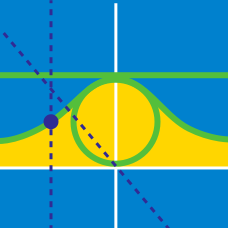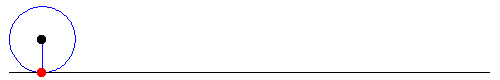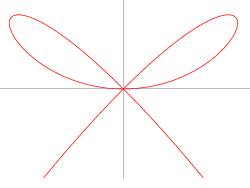Calculus

# Parametric Equations Calculus: Level 3 Challenges

If $$y$$ is a function of $$x$$ as defined by the parametric relation $$y=3\sin ^{ 2 }{ t }$$ when $$x=\tan { t }$$, then determine the value of $$\displaystyle\lim_{ x\rightarrow \infty }{ y }$$ .The parametric equation of a cycloid is given below.

$\large x = a(t - \sin t) \\ \large y = a(1 - \cos t)$

What is the area of the region bounded by the two arcs of the cycloid in the above figure?

What is the length of the arc of the curve $$x^{\frac{2}{3}} + y^{\frac{2}{3}}=4$$?The graph above satisfy the equation $$x^4 + y^3 = x^2 y$$.

The area enclosed by the 2 cute adorable little fine loops is equals to $$\frac {a}{b}$$ for coprime positive integers $$a$$ and $$b$$. What is the value of $$a+b$$?

The location of a dot $$P$$ at a given time $$t$$ in the $$xy$$ plane is given by $$(x,y) = (t - \sin t, 1 - \cos t)$$. What is the distance traveled by $$P$$ in the interval $$0 \leq t \leq 2\pi$$?

×

Problem Loading...

Note Loading...

Set Loading...